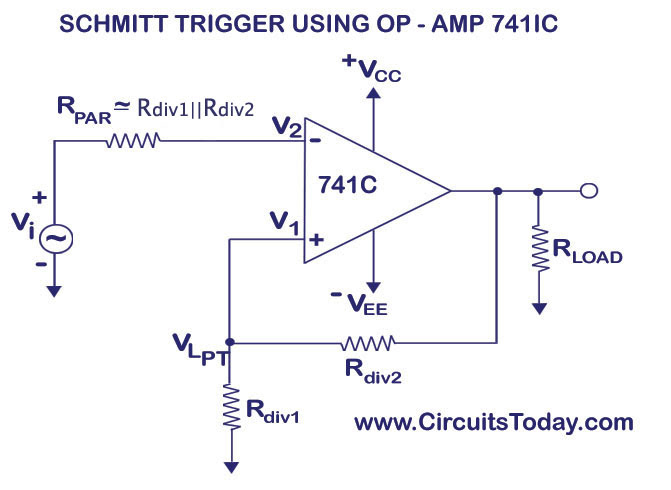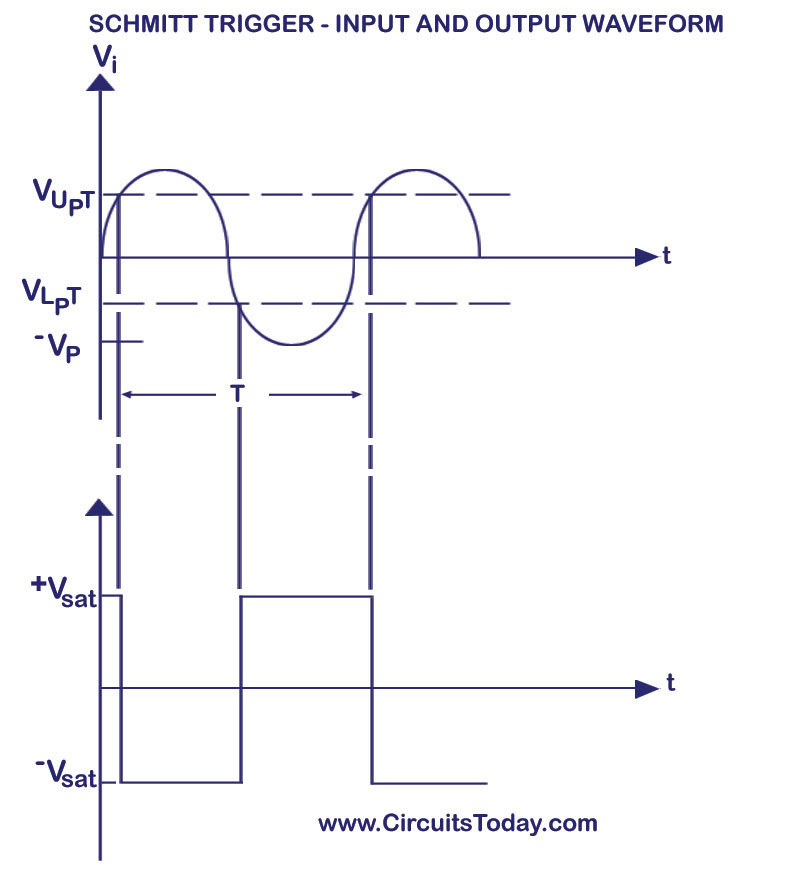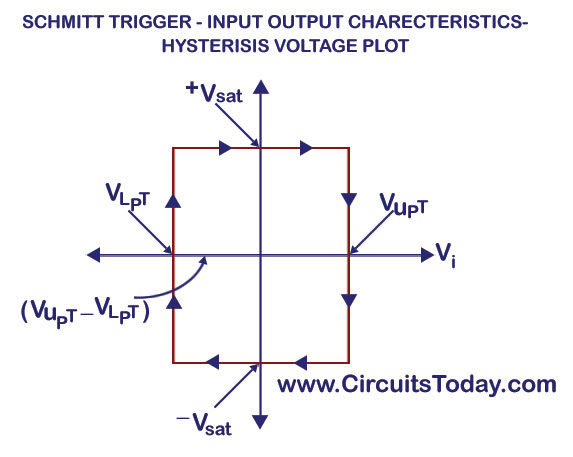## Audio

[Audio][slideshow]

### Schmitt Trigger or Regenerative Comparator Circuit

A Schmitt trigger circuit is also called a regenerative comparator circuit. The circuit is designed with a positive feedback and hence will have a regenerative action which will make the output switch levels. Also, the use of positive voltage feedback instead of a negative feedback, aids the feedback voltage to the input voltage, instead of opposing it. The use of a regenerative circuit is to remove the difficulties in a zero-crossing detector circuit due to low frequency signals and input noise voltages.

Shown below is the circuit diagram of a Schmitt trigger. It is basically an inverting comparator circuit with a positive feedback. The purpose of the Schmitt trigger is to convert any regular or irregular shaped input waveform into a square wave output voltage or pulse. Thus, it can also be called a squaring circuit.Schmitt Trigger Circuit Using Op-Amp uA741 IC

As shown in the circuit diagram, a voltage divider with resistors Rdiv1 and Rdiv2 is set in the positive feedback of the 741 IC op-amp. The same values of Rdiv1 and Rdiv2 are used to get the resistance value Rpar = Rdiv1||Rdiv2 which is connected in series with the input voltage. Rpar is used to minimize the offset problems. The voltage across R1 is fedback to the non-inverting input. The input voltage Vi triggers or changes the state of output Vout every time it exceeds its voltage levels above a certain threshold value called Upper Threshold Voltage (Vupt) and Lower Threshold Voltage (Vlpt).

Let us assume that the inverting input voltage has a slight positive value. This will cause a negative value in the output. This negative voltage is fedback to the non-inverting terminal (+) of the op-amp through the voltage divider. Thus, the value of the negative voltage that is fedback to the positive terminal becomes higher. The value of the negative voltage becomes again higher until the circuit is driven into negative saturation (-Vsat). Now, let us assume that the inverting input voltage has a slight negative value. This will cause a positive value in the output. This positive voltage is fedback to the non-inverting terminal (+) of the op-amp through the voltage divider. Thus, the value of the positive voltage that is fedback to the positive terminal becomes higher. The value of the positive voltage becomes again higher until the circuit is driven into positive saturation (+Vsat). This is why the circuit is also named a regenerative comparator circuit.Schmitt Trigger Input and Output Waveform

When Vout = +Vsat, the voltage across Rdiv1 is called Upper Threshold Voltage (Vupt). The input voltage, Vin must be slightly more positive than Vupt inorder to cause the output Vo to switch from +Vsat to -Vsat. When the input voltage is less than Vupt, the output voltage Vout is at +Vsat.

Upper Threshold Voltage, Vupt = +Vsat (Rdiv1/[Rdiv1+Rdiv2])

When Vout = -Vsat, the voltage across Rdiv1 is called Lower Threshold Voltage (Vlpt). The input voltage, Vin must be slightly more negaitive than Vlpt inorder to cause the output Vo to switch from -Vsat to +Vsat. When the input voltage is less than Vlpt, the output voltage Vout is at -Vsat.

Lower Threshold Voltage, Vlpt = -Vsat (Rdiv1/[Rdiv1+Rdiv2])

If the value of Vupt and Vlpt are higher than the input noise voltage, the positive feedback will eliminate the false output transitions. With the help of positive feedback and its regenerative behaviour, the output voltage will switch fast between the positive and negative saturation voltages.

### Hysteresis Characteristics

Since a comparator circuit with a positive feedback is used, a dead band condition hysteresis can occur in the output. When the input of the comparator has a value higher than Vupt, its output switches from +Vsat to -Vsat and reverts back to its original state, +Vsat, when the input value goes below Vlpt. This is shown in the figure below. The hysteresis voltage can be calculated as the difference between the upper and lower threshold voltages.

Vhysteresis = Vupt – Vlpt

Subsituting the values of Vupt and Vlpt from the above equations:

Vhysteresis = +Vsat (Rdiv1/Rdiv1+Rdiv2) – {-Vsat (Rdiv1/Rdiv1+Rdiv2)}

Vhysteresis = (Rdiv1/Rdiv1+Rdiv2) {+Vsat – (-Vsat)}Schmitt-Trigger-Hysteresis Characteristics

[Lights][grids]